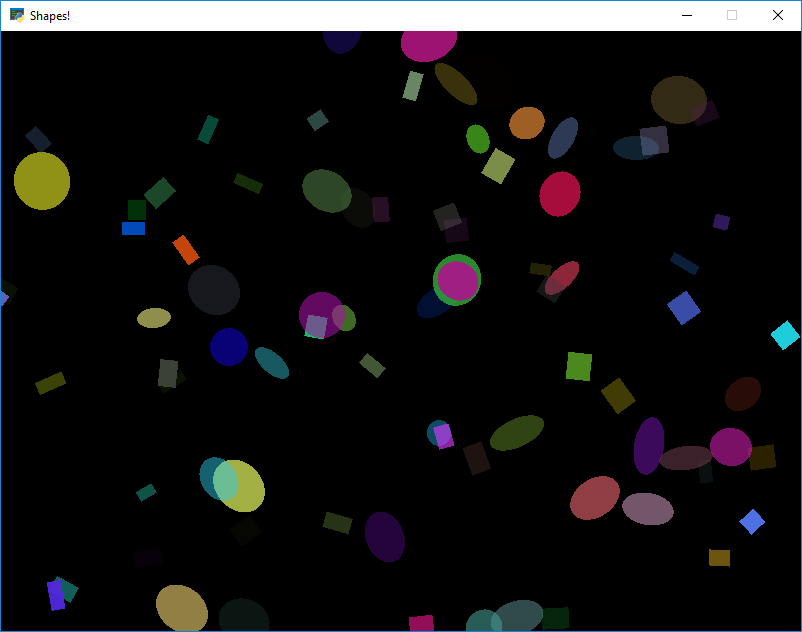# Drawing Shapes with Classes - Slowshapes.py
 ``` 1 2 3 4 5 6 7 8 9 10 11 12 13 14 15 16 17 18 19 20 21 22 23 24 25 26 27 28 29 30 31 32 33 34 35 36 37 38 39 40 41 42 43 44 45 46 47 48 49 50 51 52 53 54 55 56 57 58 59 60 61 62 63 64 65 66 67 68 69 70 71 72 73 74 75 76 77 78 79 80 81 82 83 84 85 86 87 88 89 90 91 92 93 94 95 96 97 98 99 100 101 102 103 104 105 106 107 108 109 110 111 112 113 114 115 116 117 118 119 120 121 122 123 124 125 126 127 128 129 130 131 132 133 134 135 136 137 138 139 140 141 142 143 144 145 146 147 148 149 150 151 152 153 154 155 156 157 158 159 160 161 162 163 164 165 166 167 168 169 170 171 172 173 174 175 176 177 178 179 180 181``` ```""" This simple animation example shows how to use classes to animate multiple objects on the screen at the same time. Because this is redraws the shapes from scratch each frame, this is SLOW and inefficient. Using buffered drawing commands (Vertex Buffer Objects) is a bit more complex, but faster. See https://api.arcade.academy/en/latest/examples/index.html#faster-drawing-with-shapeelementlists for this same example using shape element lists. Also, any Sprite class put in a SpriteList and drawn with the SpriteList will be drawn using Vertex Buffer Objects for better performance. If Python and Arcade are installed, this example can be run from the command line with: python -m arcade.examples.shapes_buffered """ import arcade import random import timeit # Set up the constants SCREEN_WIDTH = 800 SCREEN_HEIGHT = 600 SCREEN_TITLE = "Shapes! Non-buffered" RECT_WIDTH = 50 RECT_HEIGHT = 50 NUMBER_OF_SHAPES = 500 class Shape: """ Generic base shape class """ def __init__(self, x, y, width, height, angle, delta_x, delta_y, delta_angle, color): self.x = x self.y = y self.width = width self.height = height self.angle = angle self.delta_x = delta_x self.delta_y = delta_y self.delta_angle = delta_angle self.color = color def move(self): self.x += self.delta_x self.y += self.delta_y self.angle += self.delta_angle if self.x < 0 and self.delta_x < 0: self.delta_x *= -1 if self.y < 0 and self.delta_y < 0: self.delta_y *= -1 if self.x > SCREEN_WIDTH and self.delta_x > 0: self.delta_x *= -1 if self.y > SCREEN_HEIGHT and self.delta_y > 0: self.delta_y *= -1 class Ellipse(Shape): def draw(self): arcade.draw_ellipse_filled(self.x, self.y, self.width, self.height, self.color, self.angle) class Rectangle(Shape): def draw(self): arcade.draw_rectangle_filled(self.x, self.y, self.width, self.height, self.color, self.angle) class Line(Shape): def draw(self): arcade.draw_line(self.x, self.y, self.x + self.width, self.y + self.height, self.color, 2) class MyGame(arcade.Window): """ Main application class. """ def __init__(self): super().__init__(SCREEN_WIDTH, SCREEN_HEIGHT, SCREEN_TITLE) self.shape_list = None self.processing_time = 0 self.draw_time = 0 self.frame_count = 0 self.fps_start_timer = None self.fps = None def setup(self): """ Set up the game and initialize the variables. """ self.shape_list = [] for i in range(NUMBER_OF_SHAPES): x = random.randrange(0, SCREEN_WIDTH) y = random.randrange(0, SCREEN_HEIGHT) width = random.randrange(10, 30) height = random.randrange(10, 30) angle = random.randrange(0, 360) d_x = random.randrange(-3, 4) d_y = random.randrange(-3, 4) d_angle = random.randrange(-3, 4) red = random.randrange(256) green = random.randrange(256) blue = random.randrange(256) alpha = random.randrange(256) shape_type = random.randrange(3) # shape_type = 2 if shape_type == 0: shape = Rectangle(x, y, width, height, angle, d_x, d_y, d_angle, (red, green, blue, alpha)) elif shape_type == 1: shape = Ellipse(x, y, width, height, angle, d_x, d_y, d_angle, (red, green, blue, alpha)) elif shape_type == 2: shape = Line(x, y, width, height, angle, d_x, d_y, d_angle, (red, green, blue, alpha)) self.shape_list.append(shape) def on_update(self, dt): """ Move everything """ start_time = timeit.default_timer() for shape in self.shape_list: shape.move() self.processing_time = timeit.default_timer() - start_time def on_draw(self): """ Render the screen. """ # Start timing how long this takes draw_start_time = timeit.default_timer() if self.frame_count % 60 == 0: if self.fps_start_timer is not None: total_time = timeit.default_timer() - self.fps_start_timer self.fps = 60 / total_time self.fps_start_timer = timeit.default_timer() self.frame_count += 1 arcade.start_render() for shape in self.shape_list: shape.draw() # Display timings output = f"Processing time: {self.processing_time:.3f}" arcade.draw_text(output, 20, SCREEN_HEIGHT - 20, arcade.color.WHITE, 16) output = f"Drawing time: {self.draw_time:.3f}" arcade.draw_text(output, 20, SCREEN_HEIGHT - 40, arcade.color.WHITE, 16) if self.fps is not None: output = f"FPS: {self.fps:.0f}" arcade.draw_text(output, 20, SCREEN_HEIGHT - 60, arcade.color.WHITE, 16) self.draw_time = timeit.default_timer() - draw_start_time def main(): window = MyGame() window.setup() arcade.run() if __name__ == "__main__": main() ```